# MATHS FOR MARINERS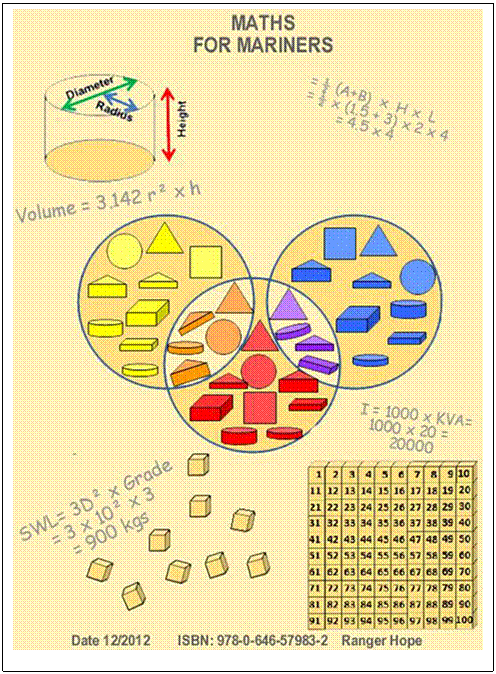This text is provided for research and study only on the understanding that users exercise due care and do not neglect any precaution which may be required by the ordinary practice of seamen or current licensing legislation with respect to its use. No copying is permitted and no liability is accepted resulting from use.

# Introduction

Chapter 4: Shape, size and capacity of tanks

4.3   Volumes of tanks

6.1   Displacement and buoyancy

6.2   Moments and longitudinal trim

7.2   Strength of rope, wire and chain

Chapter 10: Electrical calculations

# Introduction

This text is intended for those studying to begin a maritime career. It aims to jog the memory of those who may have long since left school and whose arithmetic, algebra, measure and geometry skills could be bettered.

This is a reference source for formulas and methods required to solve mathematical problems common in the mariner’s workplace. Although each section begins with basics, it is not intended to be read from start to finish. However, thumbing back to an earlier chapter may be helpful in refreshing some underpinning concepts.

I learnt arithmetic at school entirely by the rote method of daily chanting the times tables. But as schooling progressed I became entirely confounded by the mystery of slide rules, logarithms and calculus. I never really understood logic or numbers and like many I have survived with imperfect memories and a reliance on calculators. So this text is also a personal journey of re-learning of how to crawl before running. The first chapter that deals with the pre-mathematics skills of logic, sorting, base and numbering systems is provided for those who may also have been dozing at the back of the class.

# Chapter 1: Logic

## 1.1. Logic, attributes and sets

### Definitions

Logic refers to consistent rules where actions have predicable results.

Geometry describes how to draw shapes and defines the mathematical rules (relationships) concerning their construction.

Mathematics or maths describes the logical processing information using numbers.

Algebra enables logical solutions by balancing (equating) a set of calculations with a known value against one with a partially known value.

An attribute is that observable feature of a thing that makes it similar to or dissimilar from another thing. It defines what makes an individual.

To sort is to make collections (sets) of individuals determined by their common attributes.

A set is a group (collected) of individuals with one or more common attributes.

A number is a sequenced symbol used to represent an individual unit or the quantity of members of a set. The symbols we commonly use are derived from those of ancient India and Arabia. Those ancients invented the nine basic symbols of 1 representing an individual finger, 2, 3, 4, 5, 6, 7, 8, 9 representing subsequent sets of fingers (digits) and symbol 10 for the full set of fingers.

The value of a number is the size of the collection of individuals that it represents. An individual has a value of one unit.

To count is to use a systematic process to find the value of a collection. Methods have included such as using the fingers, tally marks, counters, number symbols or electronic calculators.

To process is to apply a logical rule to change an individual or to change the members of a set. In logical processes an action (a cause) has a consistent result (an effect). This predictability enables mathematics to be used to provide workplace solutions other than by trial and error.

Basic calculation is to use counters or numbers in the processes of addition (add), subtraction (take away), multiplication (times) and division (share).

### Sets and sorting

To understand our world we seek consistent rules where actions have predicable results – we look for a cause leading to a repeatable affect – we apply logic.

Our world is filled with many things - some have similar and some dissimilar features. An attribute is a defining feature of a thing. The picture below shows a collection of things that have the common attribute of being coloured wooden blocks but differing attributes of colour, shape, thickness or size. Attributes define what makes a thing an individual. For instance, there is only one circle that is red, thick and large.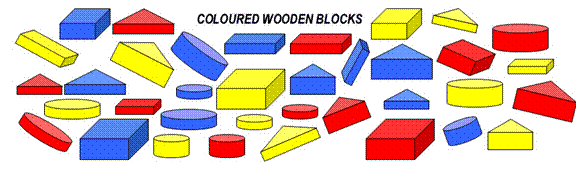Attributes allow us to compare one thing with another, make rules to classify them into logical collections and sort them into sets (groups of individuals with similar attributes). The chaotic pile of blocks above can be logically sorted and made sense of in a number of ways. All the red blocks have been selected to form a red set, leaving a remainder of those that do not have the red attribute (are yellow or blue).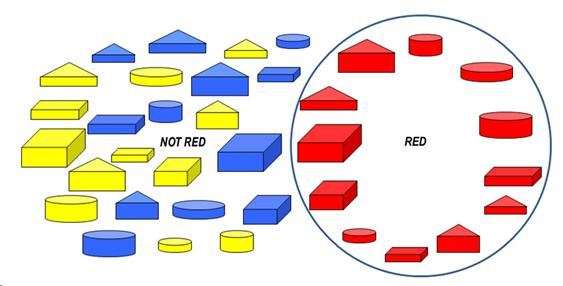However we could just as easily have created different sets by using different attributes to separate the wooden blocks into like groups. The logical rule we have used below is to select for each set on the basis of size only – large or small attribute.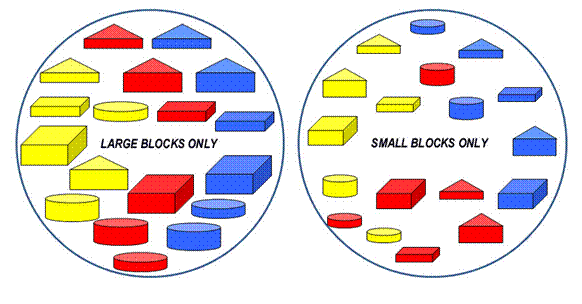Or we could have created other sets by using other attributes of the wooden blocks. The logical rule we have used below is to select on the basis of thickness only – thin or thick attribute.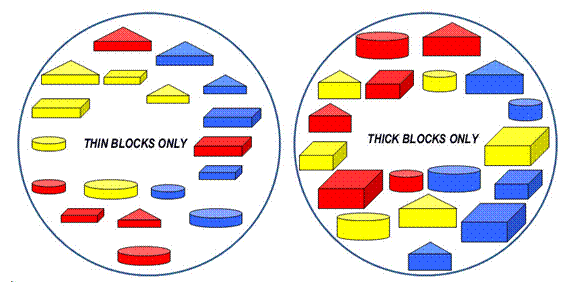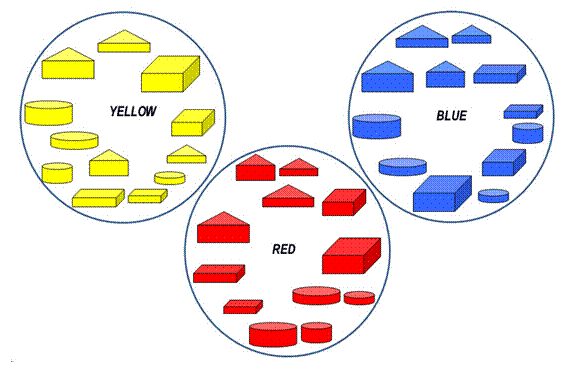Other sets are created by using the other attributes of colour or shape.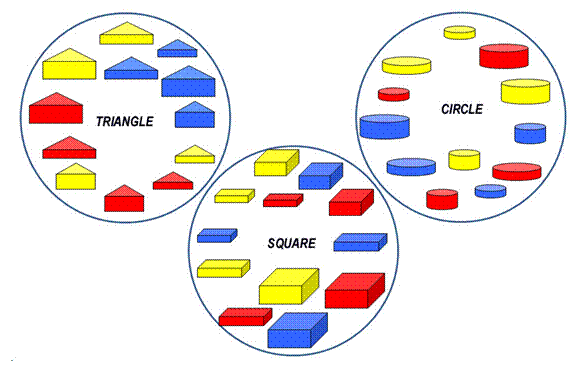In summary, attributes define what makes an individual. For instance, there is only one circle that is red, thick and large. Attributes also define the members that can make up a logical group so enabling set size to be compared. For instance, the size of each shape set above compares to a set of 10 of our fingers plus 2 of our toes.

## 1.2  Number, value, and counting

A number is a concept that represents the value of a set (collection). The number symbols used today are derived from those developed in ancient India and Arabia.

1 represents a finger or individual (a digit), 2, 3, 4, 5, 6, 7, 8, 9 represent the quantity or sequence of digits and 10 represents a full set of digits counted on both hands.

The value of a number describes the quantity of individuals in collection or the sequence of an individual’s position within a set - whether it is first, second, third etc.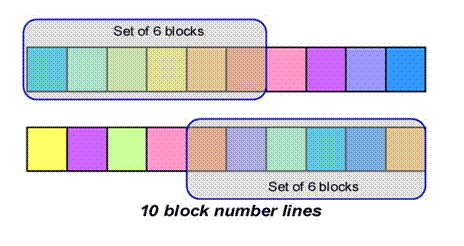Shown above are quantities of six block sets that have been separated from the number lines (each of ten blocks). Note that though both top and bottom sets contain 6 blocks, the blocks themselves are different. Number 6 is not the exclusive name of either set, but a description of a set’s attributes - they each contain six blocks.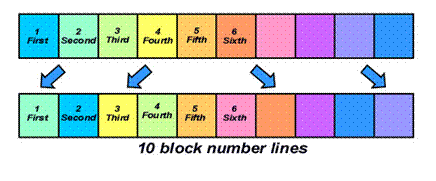Shown above are two number line sequences where the blocks have been rearranged. The name of top row yellow block number 4 is not a constant. If it is re-positioned in the bottom row then it is renamed as block number 3.

Shown below are two number line sequences where the blocks have been rearranged. This time the lower line has been reversed. Unlike the previous number lines, we can give each block a constancy of name if we apply a constancy rule - to reverse the starting point of our sequence from left to right, to right to left.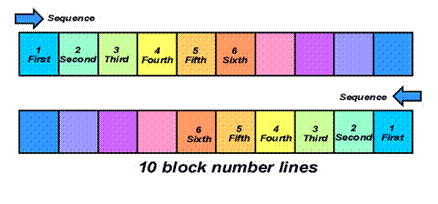It is natural to use numbers as names from an object’s place in a group, such as 1st finger, 2nd finger and 3rd finger. However, name and number are different ideas. Constancy of number relates to the logical rule not the name temporarily given to an object. An ability to trust in learnt mathematical rules and before relating back to real objects is needed in order to efficiently count, process and eventually to calculate.

To count is to use a systematic process to find the value of a collection. Methods include using the fingers, measuring, striking tally marks, use of counters, number lines, mental arithmetic, tables and electronic calculators.

### Tally - an historical perspective

With the fingers on one hand we can communicate the idea of up to five things. Counting is seen in ancient tally marks scratched onto clay tablets to represent flocks of sheep or chalked as tally marks of present day stevedores. As each collection is gathered (recorded as groups of scratches) the marks are slashed through to reckon a total - below records two sets of 5 sheep and another 4.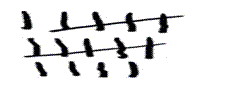Tallying requires only observation skills to accurately strike the marks, unlike processing (to apply a logical rule predicting a result). Processing is explained below.

### Processing

Once you have defined the attribute/s of an individual, a rule can be invented to process or logically change that attribute/s. The processing rule used by the colour machine shown below is to paint (change to red) anything passed through the input to the output. All other attributes remain unchanged (the shape, size and thickness).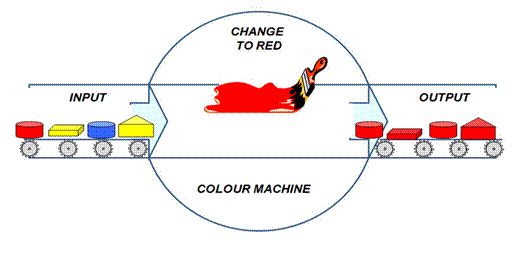However we could just as easily have created different output by changing the processing rule. The rule used by the logical thickness machine shown below is to hammer (change to thin) anything passed through the input to the output. All other attributes remain unchanged (the shape, size and colour).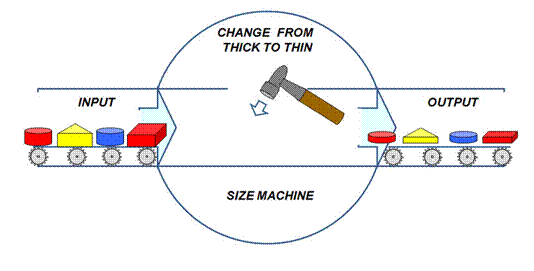Or we could just as easily have created different output by another processing rule. The rule used by the logical rounding machine shown below is to hammer (change to round) anything passed through the input to the output. All other attributes remain unchanged (the thickness, size and colour).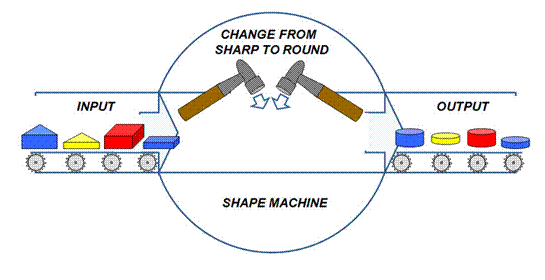Similarly the rule used by the logical copy machine shown below is to make two additional copies of anything passed through the input to the output. All attributes remain unchanged as the additional units are exact copies (clones).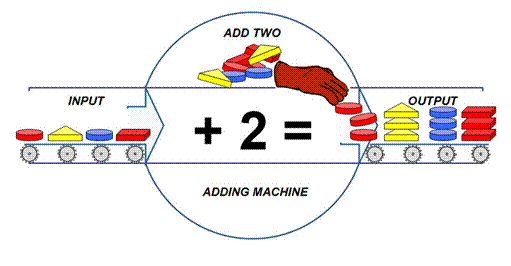We can represent this adding machine as a sum using the processing symbols of + for plus (adding) and = for equals (the result). For instance, for each block inputted:

1 + 2 = 3

1  (red square)        + 2  (red squares)            = 3  (red squares)

1  (blue circle)         + 2  (blue circles)            = 3  (blue circles)

1  (yellow triangle)  + 2  (yellow triangles)     = 3  (yellow triangles)

1  (red circle)           + 2  (red circles)              = 3  (red circles)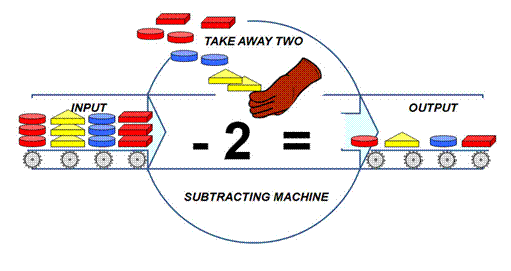Likewise the removing machine above that takes away two of anything passed through it can be represented by a subtraction using the symbols - for subtract (take away) and = for equals (the result). For instance, for each group of blocks inputted:

3 - 2 = 1

3  (red squares)           - 2  (red squares)           = 1  (red square)

3  (blue circles)           - 2  (blue circles)            = 1  (blue circle)

3  (yellow triangles)    - 2  (yellow triangles)     = 1  (yellow triangle)

3  (red circles)             - 2  (red circles)              = 1  (red circle)

The logical multiplying machine shown below that trebles anything passed through it can be represented by a multiplication using the symbols x for multiply (times) and = for equals (the answer). For instance, for each block inputted:

1 x 3 = 3

2  (yellow triangles)    x       3              = 6  (yellow triangle)

2  (blue circles)           x       3              = 6  (blue circle)

2  (red squares)          x       3              = 6  (red square)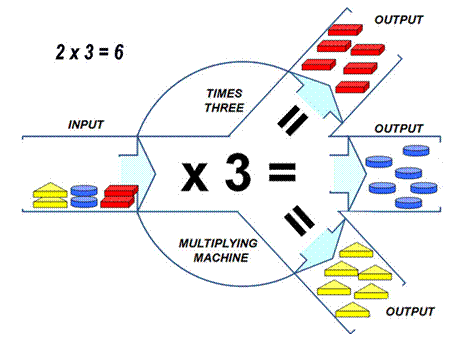The logical sharing machine shown below can be represented by a division using the symbols ÷ for divide (share) and = for equals (the answer). For instance, for each group of blocks inputted: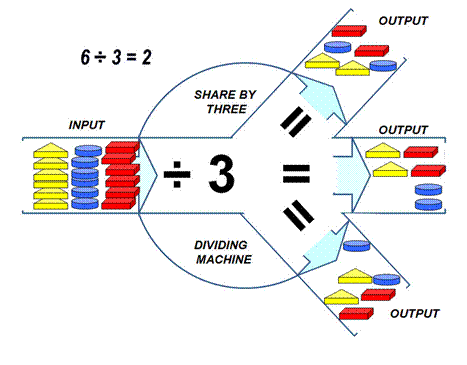### Tables and examples

 Add  + Take away  - Times  x Share  ÷ 1 + 1 =   2 1  - 1 =   0 5  - 5 =   0 1  x 1 =   1 1   ÷   1 = 1 1 + 2 =   3 2  - 1 =   1 6  - 5 =   1 1 + 3 =   4 3  - 1 =   2 7  - 5 =   2 2  x 2 =   4 2   ÷  2 = 1 1 + 4 =   5 4  - 1 =   3 8  - 5 =   3 2  x 3 =   6 4   ÷  2 = 2 1 + 5 =   6 5  - 1 =   4 9  - 5 =   4 2  x 4 =   8 6   ÷  2 = 3 1 + 6 =   7 6  - 1 =   5 2  x 5 = 10 8   ÷  2 = 4 1 + 7 =   8 7  - 1 =   6 6  - 6 =   0 10   ÷  2 = 5 1 + 8 =   9 8  - 1 =   7 7  - 6 =   1 3  x 2 =   6 1 + 9 = 10 9  - 1 =   8 8  - 6 =   2 3  x 3 =   9 3   ÷  3 = 1 9  - 6 =   3 6   ÷  3 = 2 2 + 2 =   4 2  - 2 =   0 7  - 7 =   0 9   ÷  3 = 3 2 + 3 =   5 3  - 2 =   1 8  - 7 =   1 4 x 2 =   8 2 + 4 =   6 4  - 2 =   2 9  - 7 =   2 2 + 5 =   7 5  - 2 =   3 5 x 2 =  10 4   ÷  4 = 1 2 + 6 =   8 6  - 2 =   4 8  - 8 =   0 8   ÷  4 = 2 2 + 7 =   9 7  - 2 =   5 9  - 8  =  1 2 + 8 = 10 8  - 2 =   6 9  - 9 =   0 5   ÷  5 = 1 9  - 2 =   7 10   ÷  5 = 2 3 + 3 =   6 3  - 3 =   0 10  - 1 =   9 3 + 4 =   7 4  - 3 =   1 10  - 2 =   8 3 + 5 =   8 5  - 3 =   2 10  - 3 =   7 3 + 6 =   9 6  - 3 =   3 10  - 4 =   6 3 + 7 = 10 7  - 3 =   4 10  - 5 =   5 8  - 3 =   5 10  - 6 =   4 9  - 3 =   6 10  - 7 =   3 10  - 9 =   2 4 + 4 =   8 4  - 4 =   0 10  - 9 =   1 4 + 5 =   9 5  - 4 =   1 4 + 6 = 10 6  - 4 =   2 7  - 4 =   3 5 + 5 = 10 8  - 4 =   4 9  - 4 =   5 Plus Minus Multiply Divide

## 1.3  Numbering systems and number base

All numbering systems employ a base set of symbols. The commonly used, base ten (1₁₀), imitates a full set of fingers (our digits). Combinations of the nine symbols of 1, 2, 3, 4, 5, 6, 7, 8, 9  (representing each value) and 0 (representing zero or tens of units) can represent any number. Two sets are then 20, three 30, and so on.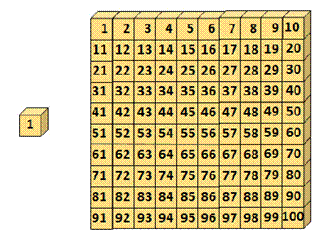Others bases are used when convenient, such as base two or binary system used in computer code. Below the tally marks show eleven marked in sets of two (Base ) and twenty three marked off in both sets of five (Base ) and in sets of ten (Base ₁₀).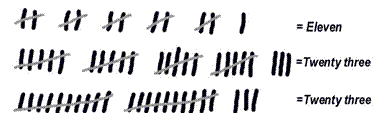### Base Five (1₅)

Base five numbering system imitates a set of fingers on one hand only and (example below) uses combinations of four symbols and a zero to express all numbers.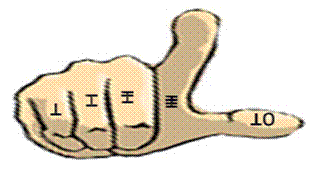In base, we have an individual digit, or we can build a square or a cube of with groups of individuals. The total value of each pile is logically numbered as shown below using the invented symbols of slashes on sticks.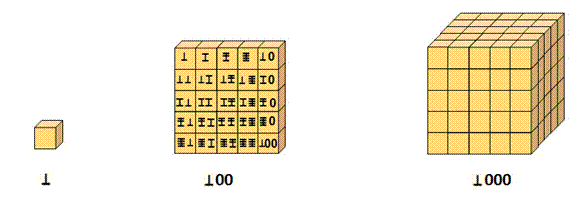In base₅ using our invented symbols we can express sets, values and a number to represent each value as: Base Sets Digits Value Number Symbol Two sets are written (digits of both hands)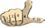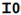Three sets are written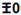Five sets are written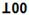However, we could have used the Indus-Arabic symbols of 1, 2, 3, 4, and 0 to express our numbers. To represent the thumb a zero is now partnered with symbol 1 so the number symbol of 10 now represents a full set of five digits.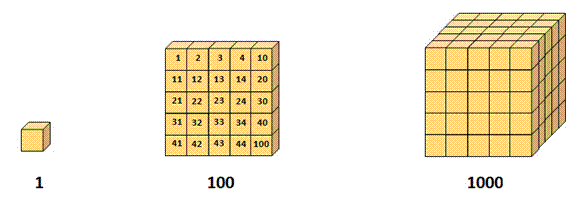Take care that you only use one base system at a time, as each base attributes a different value to its number symbols.  Base number 1000 does not have the same value as Base₁₀ number 1000₁₀.  We use base₁₀ so universaly that we rarely annotate with the base system symbol or think about the bases of our numbering systems.

 In base five using conventional Arabic symbols we can express sets, values and a number to represent each value as: Base Sets Digits Value Base 5 Base 10 Two sets are written (digits of both hands)20 10 Three sets are written30 15 Five sets are written100 25

### Base Two (1₂)

The Base-two number system (binary numeral system) is used for computer code because electronic switches can easily read and store two states, on or off (twin voltage may be used by some systems). To these states are given the symbols 1 and 0.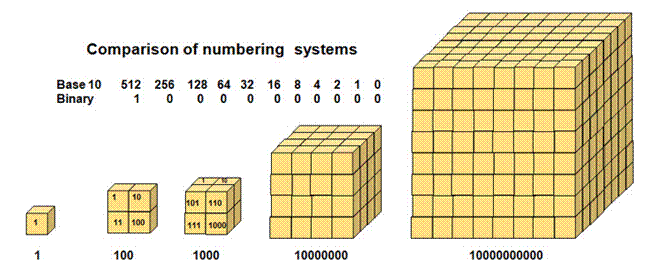A conversion from a binary number’s value to its equivalent base₁₀ value can be made with the table shown above. Binary values are derived from sets of two and progressive multiples. These values of 64, 512, 1028, and 2056 megabytes are used to specify the Random Access Memory power of a computer’s micro-processor.

## 1.4   Scientific notation - integral exponents and roots

Understand Chapter Two before reading this section. To reduce the zeros required to write the very large and very small numbers that engineers use, a short hand termed of numbers to a power (integral exponents) is used. These symbols are written by the right hand top of a number. Shown in the examples below, ten is the number and the power is to two and to three, commonly called squared or cubed.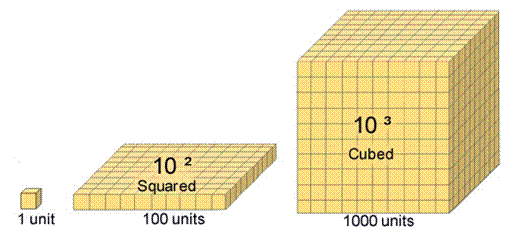Described as ten squared and written as 10² is calculated     10 x 10 =     100

Described as ten cubed and written as 10³ is calculated 10 x 10 x 10 =  1000

Similarly,

Ten to the fourth power is 10 is calculated as          10 x 10 x 10 x 10 = 10,000

Ten to the fifth power is   10 is calculated as 10 x 10 x 10 x 10 x 10 = 100,000

Any number can be written to a power as in the examples below:

Three squared is written as 3²      and calculated as                        3 x 3 =     9

Four cubed is written as             and calculated as                 4 x 4 x 4 =   32

Five to the fourth power is   5     and calculated as           5 x 5 x 5 x 5 =  625

Six to the fifth power is        6     and calculated as      6 x 6 x 6 x 6 x 6 =1296

When calculating with multiples of 10, the power defines the number of zeros (0):

Ten to the power of two has two zeros               (100 = a hundred),

Ten to the power of three has three zeros          (1000 = a thousand)

Ten to the power of six has six zeros                  (1,000,000 = a million)

Ten to the power of twelve has twelve zeros      (1,000,000,000,000 = a billion)

* Note France & US use one thousand times one million to define a US billion)

For instance, below both numbers can be written to a power.

257      =       2.57  x          100      =      2.57  x  10²

2,570,000     =        2.57 x 1,000,000      =      2.57  x  10

The convenience of exponents is further seen in expressing the concept of infinity (going on forever without end). The simple expression of ten to the power of infinity as below would otherwise require an unending task of writing 0 after 0 without end.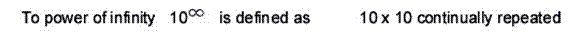Exponents also simplify otherwise tedious calculations and lessen the chance of error in the positioning of a decimal point. As below, exponents can be written as powers of ten or as decimals by use of the minus sign.

10  =  100,000                                                                     10ˉ  = 0.00001

10 =    10,000                                                                      10ˉ  = 0.0001

10³  =      1000                              10º  =  1                           10ˉ³  = 0.001

10²  =      100                                                                         10ˉ²  = 0.01

10¹  =       10                                                                          10ˉ¹  = 0.1

For consistency using decimal place, the following rule is used:

Place the decimal point immediately to the right of the first non zero digit.

Compare the number of digits between the new decimal point and the original.

This number is the value of the exponent.

0.00257      =       2.57  x         0.001      =      2.57  x  10ˉ³

0.257          =       2.57  x         0.1          =      2.57  x  10ˉ¹

### Roots (inverse powers)

The root of a number is that value that when multiplied by itself for specified repeat instances will be equal to the number. It is the inverse (reversed process) of a power, for instance: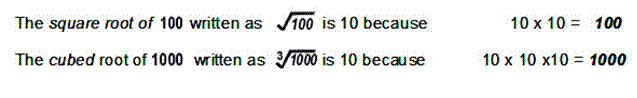All numbers have a root as in the examples below: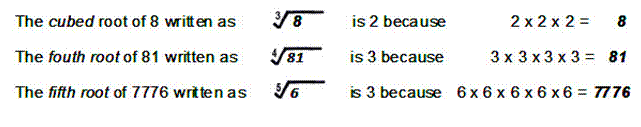Some numbers roots and number are the same, for example numbers 0 and 1 whose roots are 0 and 1: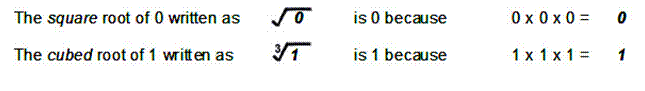The roots of some numbers are called irrational numbers because they never resolve as a whole number, for example the roots of 2, 5 and 7: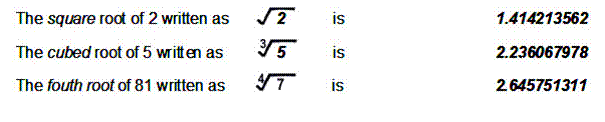### Fractional exponents

Some engineering problems require solving problems that involve the use of fractional powers. While these are easily solved following the stepped instructions with a scientific calculator, the following examples are shown in explanation.

Exponents can be written as fractions. In these cases exponent ½ indicates the power of 1 and square root of the number. Similarly exponent indicates the cube root, ¼ the fourth root, the eighth root, and so on.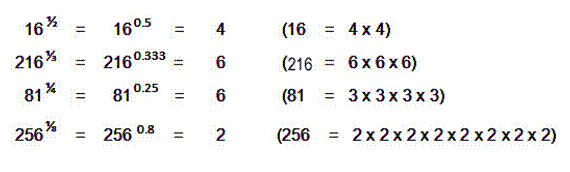In mathematical terms, the fractional exponents indicate that the numerator will raise a number to that power and the denominator takes it to that root.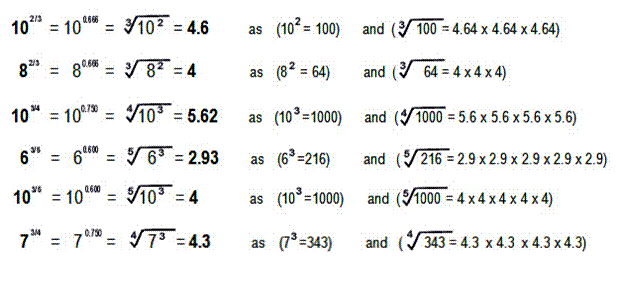# Chapter 2: Calculations

Using the fingers to count or share more than ten units is difficult. This section describes other ways to solve arithmetic problems when using base ten, the commonly used numbering system. (See more about other bases in Section 1.3).

The four principle operations are denoted by symbols (signs) and are named:

-         Minus               to subtract         or to take away       by a subtraction

x        Times               to multiply         or times                   by a multiplication

÷         Divide by         to divide            or to share               by a division

A calculation is a arithmetical problem with its solution (its answer). Calculations can be expressed (written down in maths shorthand) using an equation. The problem is written on the left, the solution on the right with an equals symbol (=) between them. In a balanced equation, each side of the equals sign must have the same total. (See more on equations and algebra in Section 2.4). Simple equations are shown below:

1 + 1 = 2            or           3 - 1 = 2         or         2 x 2 = 4        or         4 ÷ 2 = 2

1 plus 1 equals 2         3 minus 1 equals 2            2 times 2 equals 4           4 divide by 2 equals 2

## Various methods used to find solutions are descibed below.

### Using the Number Line method and mental arithmetic

A scale or ruler is a number line when used as a visual aid in addition (counting out), subtraction (finding a remainder), division (sharing) and multiplication (times).

In your mind cut the stick below into 12 slices and then count the slices out to share with ten persons. Ten can be evenly shared but two slices are left over. We have used the number line below to record our sharing and the remainder that was left.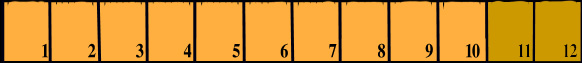What we have done can be expressed by the equations:

1+1+1+1+1+1+1+1+1+1=10 shared orange             12 -10 = 2 remainder brown

Multiplication and division could also be visualised using the number line. The 12 slices could be counted out in sets (See 1.1 Sets and sorting):

2 sets of      six slices can be written as           2 x 6 = 12      or         12 ÷ 6 = 2

3 sets of      four slices can be written as         3 x 4 = 12      or         12 ÷ 3 = 4

4 sets of      three slices can be written as       4 x 3 = 12      or         12 ÷ 4 = 3

6 sets of      two slices can be written as          6 x 2 = 12      or         12 ÷ 2 = 6

While this scale is marked in twelve units, each unit could be further divided into 8 sub units to solve more complex problems (by counting up the units), as below:

1 units            x             8 sub-units            =        8 sub-units

4 units            x             8 sub-units            =       32 sub-units

12 units            x             8 sub-units            =       96 sub-unitsThe number line works well for small numbers but as it relies on counting out every unit it is slow for complex problems.

### Using the Times Tables method

Fast solution to maths problems can be looked up or learnt as the times tables below. (also see addition tables Section1.2). They can also be used to find division solutions. It can be seen that one side of each equation is equal to the other. For example, in the times x 4 table equation of below 8 x 4 = 32, it is also true that 32 shared among 4 will have a solution of 8.

8 x 4 = 32             or        32 ÷ 4 = 8

 Times x 1 Times x 2 Times x 3 Times x 4 Times x 5 Times x 6 1 x 1 =  1 1 x 2 =   2 1 x 3 =   3 1 x 4 =   4 1 x 5 =   5 1 x 6 =   6 2 x 1 =  2 2 x 2 =   4 2 x 3 =   6 2 x 4 =   8 2 x 5 = 10 2 x 6 = 12 3 x 1 =  3 3 x 2 =   6 3 x 3 =   9 3 x 4 = 12 3 x 5 = 15 3 x 6 = 18 4 x 1 =  4 4 x 2 =   8 4 x 3 = 12 4 x 4 = 16 4 x 5 = 20 4 x 6 = 24 5 x 1 =  5 5 x 2 = 10 5 x 3 = 15 5 x 4 = 20 5 x 5 = 25 5 x 6 = 30 6 x 1 =  6 6 x 2 = 12 6 x 3 = 18 6 x 4 = 24 6 x 5 = 30 6 x 6 = 36 7 x 1 =  7 7 x 2 = 14 7 x 3 = 21 7 x 4 = 28 7 x 5 = 35 7 x 6 = 42 8 x 1 =  8 8 x 2 = 16 8 x 3 = 24 8 x 4 = 32 8 x 5 = 40 8 x 6 = 48 9 x 1 =  9 9 x 2 = 18 9 x 3 = 27 9 x 4 = 36 9 x 5 = 45 9 x 6 = 54 10 x 1 =10 10 x 2 = 20 10 x 3 = 30 10 x 4 = 40 10 x 5 = 50 10 x 6 = 60 Times x 7 Times x 8 Times x 9 Times x 10 Times x 11 Times x 12 1 x 7 =   7 1 x 8 =   8 1 x 9 =  9 1 x 10 = 10 1 x 11 = 11 1 x 12 = 12 2 x 7 = 14 2 x 8 = 16 2 x 9 = 18 2 x 10 = 20 2 x 11 = 22 2 x 12 = 24 3 x 7 = 21 3 x 8 = 24 3 x 9 = 27 3 x 10 = 30 3 x 11 = 33 3 x 12 = 36 4 x 7 = 28 4 x 8 = 32 4 x 9 = 36 4 x 10 = 40 4 x 11 = 44 4 x 12 = 48 5 x 7 = 35 5 x 8 = 40 5 x 9 = 45 5 x 10 = 50 5 x 11 = 55 5 x 12 = 60 6 x 7 = 42 6 x 8 = 48 6 x 9 = 54 6 x 10 = 60 6 x 11 = 66 6 x 12 = 72 7 x 7 = 49 7 x 8 = 56 7 x 9 = 63 7 x 10 = 70 7 x 11 = 77 7 x 12 = 84 8 x 7 = 56 8 x 8 = 64 8 x 9 = 72 8 x 10 = 80 8 x 11 = 88 8 x 12 = 96 9 x 7 = 63 9 x 8 = 72 9 x 9 = 81 9 x 10 = 90 9 x 11 = 99 9 x 12 =108 10 x 7 = 70 10 x 8 = 80 10 x 9 = 90 10 x 10 =100 10 x 11 =110 10 x 12 =120

### Using Short calculations - simple addition, subtraction, multiplication, division

Short addition calculations are written as columns to sum in an answer box below:

 UNIT UNIT UNIT UNIT 5 4 7 6 +3 +5 +2 +3 8 9 9 9

Lists can also be added. Note - for numbers of 10 or more the first step is to add the Units column (right hand in blue below), and the second step the left hand Tens:

 TENS  UNITS TENS  UNITS 3 4 4 1 2 2 1 1 2 1 3 +3 +2 +1 2 +1 0 8 7 2 8 3 5

 2 4 5 2 1 1 1 3 +2 +2 +3 +5 ? ? ? ?? 5 6 12 21 5 10 14 13 +5 +12 +10 +15 ?? ?? ?? ?? Answers addition - as below: 2 4 5 2 1 1 1 3 +2 +2 +3 +5 5 7 9 10 5 6 12 21 5 10 14 13 +5 +12 +10 +15 15 28 36 49

Short subtraction calculations can also be written in the same format as below. Note - for numbers of 10 or more the first step is to add the Units column (U), and the second step adds the Tens column (T):

 T  U T U 5 6 17 32 -3 -5 -12 -11 2 1 5 21

Check your progress subtraction -try these calculations:

 T U T U 6 5 15 25 -2 -3 - 3 -12 ? ? ?? ?? Answers subtraction - as below: 6 5 15 25 -2 -3 - 3 -12 4 2 12 13

Short multiplication calculations can be written as below. If we have 10 or more on the top line we multiply the Units first (U) and the Tens last (T):

 T  U T  U 3 4 14 12 x 2 x 3 x 2 x 4 6 12 28 48

Check your progress multiplication -try these calculations:

 T U T U 5 12 24 33 x 2 x 3 x 2 x 3 ?? ?? ?? ?? Answers multiplication - as below: 5 12 24 33 x 2 x  3 x  2 x  3 10 36 48 99

Short division calculations are written differently. The divisor (how many to share with) is written on the left while he quantity to share is boxed off to the right. The answer is written above the division box (in blue above).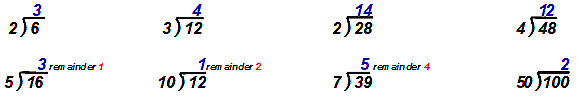If the quantity does not share equally we write down a remainder (in red above)

 Check your progress division -try these calculations: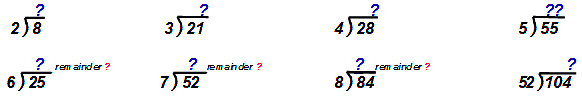Answers division - as below: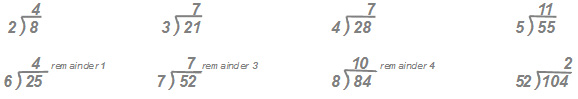#### Using Long calculations - addition, subtraction, multiplication and division

Long addition calculations - With larger numbers the Unit columns can add up to ten or more. To solve this kind of problem we first add the Unit column (blue) but in the answer box only write the unit part of the answer. We carry over the ten value to the Ten’s column, then sum that column with the carried over as the full answer.

In example one, the step one adds the units column 5 + 7 = 12. We write the 2 from the 12 in our answer box and then pencil in 1 (shown red) next to column of tens. We then add the new tens column 1 ten + 2 tens + the carried over ten and find the result is 4 lots of ten. The solution is 42. For larger sums we may need a step three with a Hundreds column (H).

 Example : Example : Step 1 Step 2 Step 1 Step 2 T  U T  U T  U T  U 1 5 ¹1 5 3 5 ¹3 5 +2 7 +2 7 +4 9 +4 9 ? 2 4 2 ? 4 8 4 Example : Example : Step 1 Step 2 & 3 Step 1 Step 2 & 3 H  T  U H  T  U H  T  U H  T  U 2 1 5 2¹1 5 3 6 5 ¹4¹6 5 +4 2 7 +4 2 7 +5 8 9 +5 8 9 ? 3 2 6 4 2 ? ? ? 4 1 0 5 4

 Check your progress with long addition sums - try these calculations: Question 1: Question  2: Step 1 Step 1 T  U T  U 5 6 7 8 +3 9 +5 9 ? ? ? ? Question  3: Question  4: Step 1 Step 1 H  T  U H  T  U 6 3 5 4 6 5 +2 6 7 +5 8 9 ? ? ? ? ? ? ? Answers  with long addition sums - as below: - try these calculations: Answer 1: Answer  2: Step 1 Step 2 Step 1 Step 2 T  U T  U T  U T  U 5 6 ¹5 6 7 8 ¹7 8 +3 9 +3 9 +5 9 +5 9 ? 5 9 5 ? 7 1 3 7 Answer  3: Answer  4: Step 1 Step 2 & 3 Step 1 Step 2 & 3 H  T  U H  T  U H  T  U H  T  U 6 3 5 ¹6¹3 5 4 6 5 ¹4¹6 5 +2 6 7 +2 6 7 +5 8 9 +5 8 9 ? ? 2 9 0 2 ? ? ? 4 1 0 5 4

Long subtraction calculations – either of two methods described below are used:

First method - When the top line units are greater than the bottom line, we have to borrow from the next greater column (tens or hundreds). The first Step borrows from the ten column by pencilling 1 (ten) next in the unit’s column enabling completion of the units part of the answer. To pay back the borrowed ten we cross out the bottom ten’s numeral and add to it a ten payback correction.

In example nine, subtracting the units 5 - 6 not possible, so the first Step is to borrow as 15 – 6 = 9.  But the ten has to be paid back so in Step two the 2 (tens) bottom numeral is crossed and replaced with 3, so enabling the solution of 19 to be written in the answer box. In example eleven we have to borrow from both the ten’s and from the hundred’s column to get our answer.

 Example : Example : Step 1 Step 2 Step 1 Step 2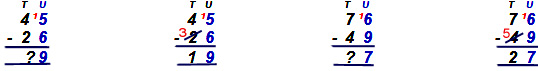Example : Step 1 Step 2 Step 3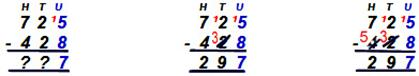Example : Step 1 Step 2 Step 3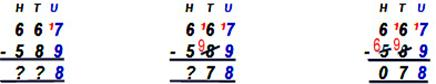Note in example twelve the hundreds column of 6 hundreds minus 6 hundreds gives an answer of zero hundreds (0). If the taking away number is larger than the number to be taken away from then the arrangement above will not work. In such cases we reverse position of the lines so we can continue taking away a smaller number from a larger, but call the answer we call a minus number.   See example thirteen where we have 637- 834 = -197. For our calculation to work we must take away 637 (the smaller) from 834 (the larger) and call the answer a minus number (-197) Example : Step 1 Step 2 Step 3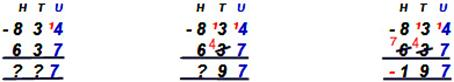Long subtraction calculations

Second method - When the top line units are greater than the bottom line, we have to borrow from a higher column. The first Step borrows from the ten’s column by pencilling 1 (ten) next in the top line unit so enabling completion of the units part of the answer. To pay back the borrowed ten we cross out top line ten’s numeral and subtract a ten from it for a payback correction.

In example fourteen, Step One finds subtracting the units 5 - 6 not possible, so ten is borrowed to calculate 15 – 6 = 9.  But the ten has to be paid back so in Step Two the 4 (tens) top numeral is crossed out and replaced with ten less (3), so enabling the answer box to be completed with the same solution as the first method of 19. In example sixteen we have to borrow from the ten’s and from the hundred’s column to get our answer.

 Example : Example : Step 1 Step 2 Step 1 Step 2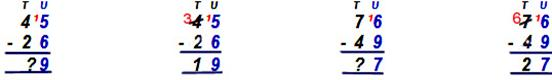Example : Step 1 Step 2 Step 3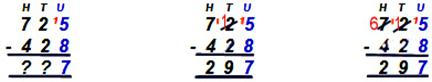Example : Step 1 Step 2 Step 3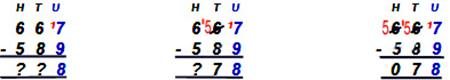Check your progress with long subtraction - try these calculations: Question 1: Question 2: T    U T    U 7  7 4  2 -  5  8 -  3  9 ? ? ?  ? Question 3: Question 4: H   T   U H   T   U 6  2  3 8  5  3 -  4  8  9 -  5  6  5 ?  ?  ? ?  ?  ?

 Answers long subtraction: Answer 1: Answer 2: Step 1 Step 2 Step 1 Step 2 T    U T   U T    U T    U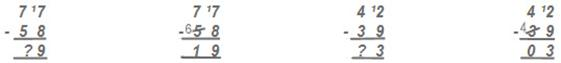Answer 3: Step 1 Step 2 Step 3 H   T   U H    T   U H   T    U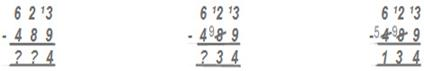Answer 4: Step 1 Step 2 Step 3 H   T   U H   T   U H   T   U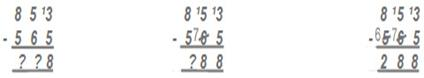Long multiplication calculations – Times tables are not readily available for multipliers of over twelve, so greater calculations are done in steps. The first Step is to multiply the units, then the tens and then hundreds. Finally all the answer lines are added. Remember to add a zero (0) as will be required for the ten line to be the correct value, as in Example 18 below:

 Example : Step 1 Step 2 Step 3 Step 4 H   T  U H   T  U H   T  U H   T  U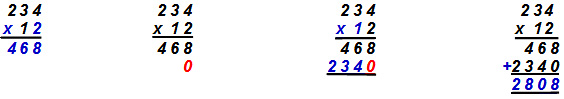Example : Step 1 Step 2 Step 3 Step 4 H   T  U H   T  U H  T  U T  H  T  U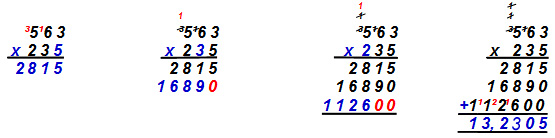Check your progress multiplication -try these calculations: Question 1: Question 2: Question 3: Question 4: 4 2 3 2 3 7 5 6 8 7 7 7 x  2 5 x  5 2 x 3 1 2 x 4 3 6 ? ? ? ? ? ? ? ? ? ? ? ? Answers multiplication - as below: Answer 1: Answer 2: Answer 3: Answer 4: 4 2 3 2 3 7 5 6 8 7 7 7 x  2 5 x  5 2 x 3 1 2 x 4 3 6 2 1 1 5 4 7 4 1 1 3 6 4 6 6 2 8 4 6 0 1 1 8 5 0 5 6 8 0 2 3 3 1 0 1 0,5 7 5 1 2,3 2 4 1 7 0 4 0 0 3 1 0 8 0 0 1 7 7,2 1 6 3 3 8 7 7 2

Long division calculations – In example 20 below, Step 1 estimates how many divisors (5) fit in the left hand boxed numeral (1). If it will not fit, Step 2 estimates how many fit into the first + the second numerals (1 & 3), in the example giving 2. As 2 x 5 =10 we subtract 10 from 1 & 3 to leave 3.  Step 3 drops the third numeral (5) to give 35. Step 4 finds that the divisor fits 7 times into 35 giving a final answer of 27.

 Example : Step 1 Step 2 Step 3 Step 4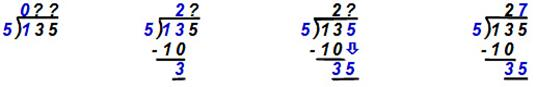Example : Step 1 Step 2 Step 3 Step 4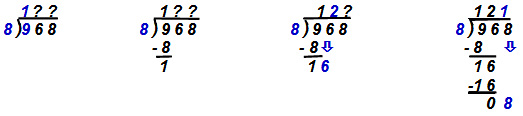Example : Step 1 Step 2 Step 3 Step 4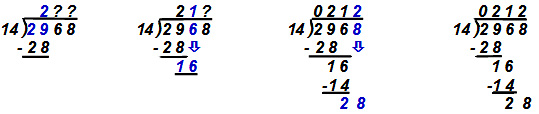Example : Step 1 Step 2 Step 3 Step 4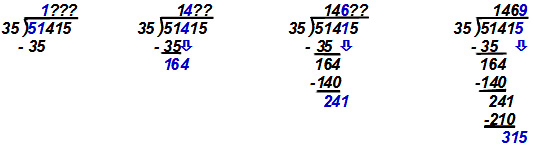Example : If the quantity does not share equally we write down a remainder (in red below), or write it as a fraction (see next Section 2.3) Step 1 Step 2 Step 3 Step 4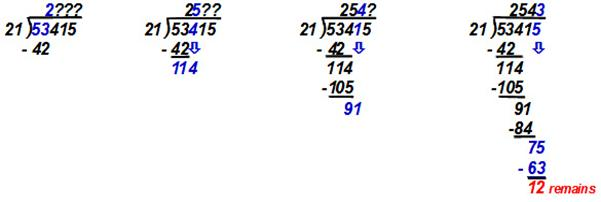Check your progress long division -try these calculations: Question 1: Question 2: Question 3: Question 4: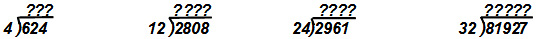Answers long division - as below: Answer 1: Answer 2: Answer 3: Answer 4: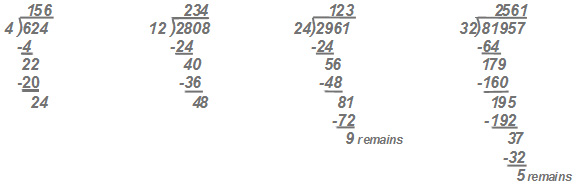## 2.3   Fractions and decimals

### Fractions

To share a cake we slice it into parts. Each part can be called a fraction of the whole cake, for example, slice number 1 below is from a cake with 8 slices. It is one eighth of the cake. All fractions are parts of a whole.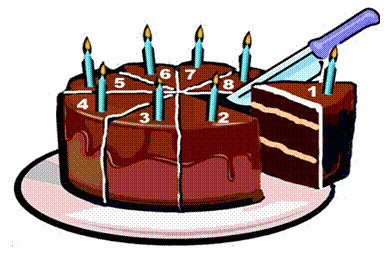Fraction’s are expressed (written) separated by a line – as above 1 of a cake. 8

A numerator -       a number on top showing us the parts of the whole we have.

A denominator -   a bottom number showing how many parts make up the whole.

The cake could have been cut into any other fractions, such as:

 1 = one slice of a whole cake cut into two = half a cake 2 1 = one slice of a whole cake cut into four = quarter of a cake 4 1 = one slice of a whole cake cut into ten = tenth of a cake 10

#### Calculating with fractions

Addition – with a common denominator

To add fractions the denominators (the number below the line) must be the same (called common). The answer is found by totalling the numerators (number above the line) of each fraction and expressing with the common denominator, as below:

 1 + 2 + 3 + 4 = 10 half a 20 20 20 20 20 cake Expression and Substitution However, we could have received several cake slices expressed as fractions below: 4 = four slices of a whole cake cut into eight = 1 half of cake 8 2 4 = four slices of a whole cake cut into twelve = 1 third of cake 12 3

You will notice that both 4 slices of an 8 slice cake and10 slices of a 20 slice cake both are half of each cake. So fractions can be expressed with differing numerator and denominator and still be the same value as a part of the whole, for instance:

 10 = 1 = 5 = 2 = 10 20 2 10 4 20

The rule is that we must change both the top and the bottom line of a fraction with the same factor (divisor or multiplier) if the fraction is to retain its value. Applying this rule is called substitution.

 10         x 4 = 40    ÷ 10 = 4   x 3 = 12     ÷ 12 = 1 ü 20        x 4 80    ÷ 10 8   x 3 24     ÷ 12 2 Note below that substitution does not work by adding or subtracting to numerator and denominator. Only by multiplying and dividing equally gives a correct answer. 10      + 4 = 14 X Wrong!! 10      - 4 = 6 X Wrong !! 20      + 4 24 20      - 4 16

Addition – fractions without a common denominator

We need a common denominator to add fractions. But in the example below different cakes have been cut into different numbers of slices. Each cake’s denominator is different. We have to change each fraction to the same denominator but still express its same value (part of a whole cake). All fractions must be of same sized slices.

To find our common denominator we could multiply all denominators, as below:

 10 + 3 + 6 + 2 = ? 20 5 10 4 ? 20 x 5 x 10 x 4 = 4000

The common denominator in this case is 4000, a very large number of tiny slices of a cake and a complex number for calculations. It will be easier for us to find the lowest common denominator, the smallest number that all denominators will divide into without leaving any remainder. In the example below it is 20.

Step 1 -  draw a long line above our chosen lowest common denominator 20.

Step 2 -  divide the lowest common denominator with each fraction’s denominator.

Step 3 -  multiply the answer with each old numerator. Mathematical balance is regained with the value being equal to each old fraction’s value. Finally total the numerators and express the answer with the lowest common denominator.

 10 + 4 + 6 + 2 = ? 20 5 10 4 ? Step 3: 1 x 10 = 20 4 x 4 =  12 2  x  6 = 12 5 x 2 = 10 Step 2: 20 ÷ 20 =  1 20 ÷ 5 =  4 20 ÷ 10 =  2 20 ÷ 4 =  5 Step 1: 10    +    16    +   12     +   10 = 48 = 2 8 = 2 2 20 20 20 5

Unlike earlier examples our answer is an improper fraction. The numerator 48 is greater than the denominator 20.  Remember, the slices are from more than one cake. To express properly we divide the numerator with the denominator. The 48 pieces from 20 sliced cakes could be reassembled as 2 wholes with 8 slices left over. Eight twentieths can be substituted as the same as two fifths of a cake.

Sometimes we have to add whole numbers with fractions as below. The method is to convert each whole number with fraction into an improper fraction, find the common denominator and then continue as normal addition. The answer, an improper fraction, is converted back to whole number with any remainder fraction. The remainder can be substituted with lowest denominator for the simplest expression.

 1 1 + 2 2 + 1 1 = ? 2 3 6 2 + 1 + 6 + 2 + 6 + 1 = ?